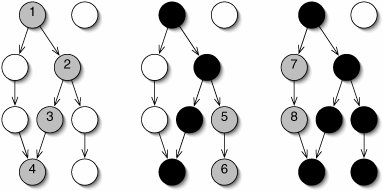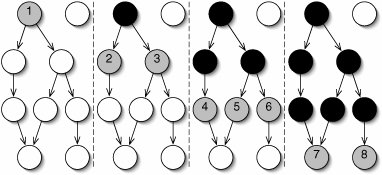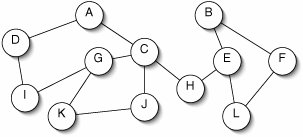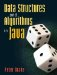# Graph Traversal

Like a tree, a graph can be traversed in more than one way. We must choose an arbitrary vertex to start from. This is called the source vertex. A depth-first traversal (Figure 15-18) follows edges until it reaches a dead end, then backtracks to the last branching point to try a different branch. The order in which the different branches are tried is arbitrary.

Figure 15-18. Depth-first traversal of a graph. The traversal begins by following edges until a dead end is reached (left). It then backtracks to the last decision point, following a different branch (middle). This continues until all reachable vertices have been visited (right).

(This item is displayed on page 420 in the print version)A breadth-first traversal (Figure 15-19) visits one vertex, then its neighbors, then vertices two edges away, and so on.

Figure 15-19. In a breadth-first traversal of a graph, vertices closer to the source vertex are visited earlier than more distant vertices. The dashed lines merely separate the four copies of the graph.

(This item is displayed on page 420 in the print version)In either case, vertices that cannot be reached from the source (such as the one at the upper right in Figures 15-18 and 15-19) are never visited. Because there may be more than one path to a given vertex, it is necessary to keep track of which vertices have already been visited. This is done with an array of booleans.

The algorithm for depth-first traversal can be stated using a stack or, more concisely, using recursion (Figure 15-20).

Figure 15-20. Depth-first traversal of a graph. The second, protected method depthFirstTraverse() is recursive.

 1 /** 2 * Return a list of the vertices reachable from source, in depth- 3 * first order. 4 */ 5 public List depthFirstTraverse(int source) { 6 List result = new ArrayList(size()); 7 boolean[] visited = new boolean[size()]; 8 depthFirstTraverse(source, result, visited); 9 return result; 10 } 11 12 /** 13 * Visit the vertices reachable from vertex, in depth-first order. 14 * Add vertices to result as they are visited. 15 */ 16 protected void depthFirstTraverse(int vertex, 17 List result, 18 boolean[] visited) { 19 visited[vertex] = true; 20 result.add(vertex); 21 for (Integer i : neighbors(vertex)) { 22 if (!visited[i]) { 23 depthFirstTraverse(i, result, visited); 24 } 25 } 26 }

The algorithm for breadth-first traversal (Figure 15-21) uses a queue, much like the level order traversal of a tree (Section 10.2).

Figure 15-21. Breadth-first traversal of a graph.

 ``` 1 /** 2 * Return a list of the vertices reachable from source, in 3 * breadth-first order. 4 */ 5 public List breadthFirstTraverse(int source) { 6 List result = new ArrayList(size()); 7 boolean[] visited = new boolean[size()]; 8 Queue q = new LinkedList(); 9 visited[source] = true; 10 q.offer(source); 11 while (!(q.isEmpty())) { 12 int vertex = q.poll(); 13 result.add(vertex); 14 for (Integer i : neighbors(vertex)) { 15 if (!visited[i]) { 16 visited[i] = true; 17 q.offer(i); 18 } 19 } 20 } 21 return result; 22 } ```

Exercises

 15.12 Figure 15-22 shows an undirected graph. In what order might the vertices be visited during a depth-first traversal starting at vertex G? What about a breadth-first traversal? (There is more than one correct answer for each question.)

Figure 15-22. Undirected graph for Exercise 15.1215.13 What bad thing could happen if we removed the test on line 22 of Figure 15-20?

### Topological SortingData Structures and Algorithms in Java
ISBN: 0131469142
EAN: 2147483647
Year: 2004
Pages: 216
Authors: Peter Drake